## Helix

A helix is also called a Curve of Constant Slope. It can be defined as a curve for which the Tangent makes a constant Angle with a fixed line. The helix is a Space Curve with parametric equations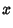(1)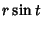(2)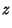(3)

whereand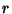are constants. The Curvature of the helix is given by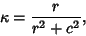(4)

and the Locus of the centers of Curvature of a helix is another helix. The Arc Length is given by(5)

The Torsion of a helix is given by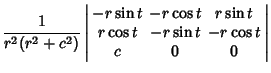(6)

so(7)

which is a constant. In fact, Lancret's Theorem states that a Necessary and Sufficient condition for a curve to be a helix is that the ratio of Curvature to Torsion be constant. The Osculating Plane of the helix is given by(8)(9)

The Minimal Surface of a helix is a Helicoid.

References

Geometry Center. The Helix.'' http://www.geom.umn.edu/zoo/diffgeom/surfspace/helicoid/helix.html.

Gray, A. The Helix and Its Generalizations.'' §7.5 in Modern Differential Geometry of Curves and Surfaces. Boca Raton, FL: CRC Press, pp. 138-140, 1993.

Isenberg, C. Plate 4.11 in The Science of Soap Films and Soap Bubbles. New York: Dover, 1992.

Pappas, T. The Helix--Mathematics & Genetics.'' The Joy of Mathematics. San Carlos, CA: Wide World Publ./Tetra, pp. 166-168, 1989.

Wolfram, S. The Mathematica Book, 3rd ed. Champaign, IL: Wolfram Media, p. 163, 1996.